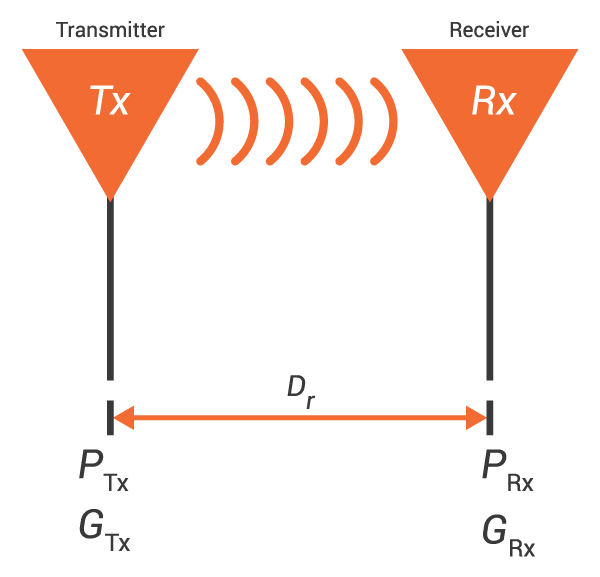# Friis Transmission Calculator

## This calculator computes the power received by an antenna using Friis formula

(dBi)
(dBi)

milliwatts (mW)

### Overview

The Friis Equation is used to find the ideal power arriving at the receiving antenna given the transmitted power, the signal frequency, travel distance and the gains of the antenna. To use this calculator, just enter the required parameters and press the "calculate" button.### Equation

$$P_{RX} = P_{TX}G_{TX}G_{RX}(\frac{c}{4 \pi D f})^2$$

Where:

$$P_{RX}$$ = power arriving at the receiver (watts)

$$P_{TX}$$ = power at the transmitter (watts)

$$G_{TX}$$ = gain at the transmitter (absolute)

$$G_{RX}$$ = gain at the receiver (absolute)

$$c$$ = speed of light  = 3 x 108 m/s

$$D$$ = distance between transmitter and receiver (meters)

$$f$$ = signal frequency (hertz)

### Applications

Friis equation is useful in calculating the signal power arriving at the receiver. However in actual situations, a signal is composed of different frequencies whereas Friis equation only uses one frequency. Friis equation provides a good approximation of the received signal power if the center frequency is high enough. This is because the difference in received power over the bandwidth at a high center frequency is too small to be significant.

Textbook - Principles of Radio: Basic AC Theory

Technical Article - Fundamentals of Wi-Fi Antennas

Worksheet - Design Project: Radio Transmitter

1 Comment• M
Mr ' June 10, 2022

I think there is an error in this calculator as it gives a different answer to these two:

To me it looks like the antenna gains are not being converted from dBi to absolute ratios before being put into the calculation.  Increasing the gain of either antenna by 10dBi should increase the received power by a factor of 10, but going from 10dBi to 20dBi in this alcualtor only doubles the received power.

Like.